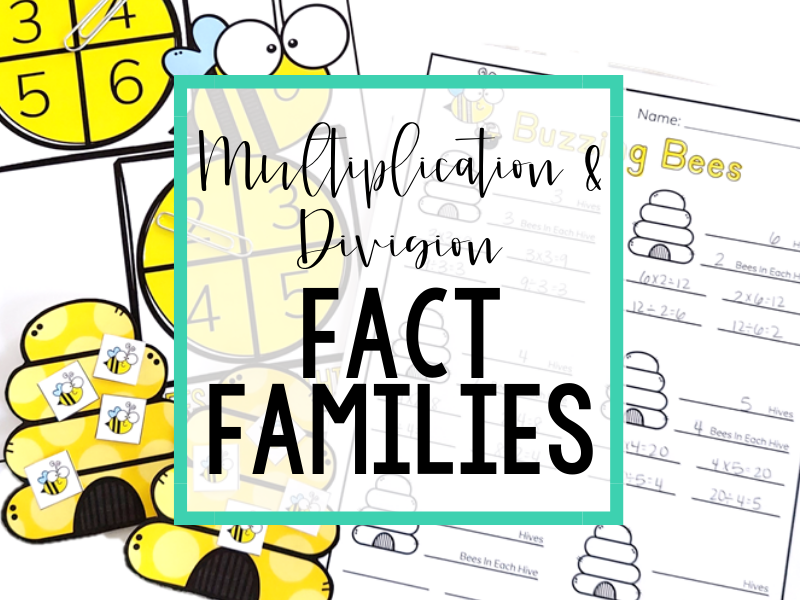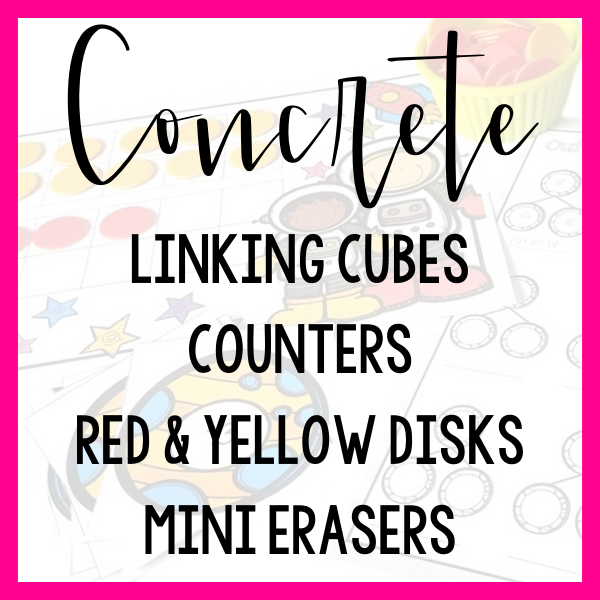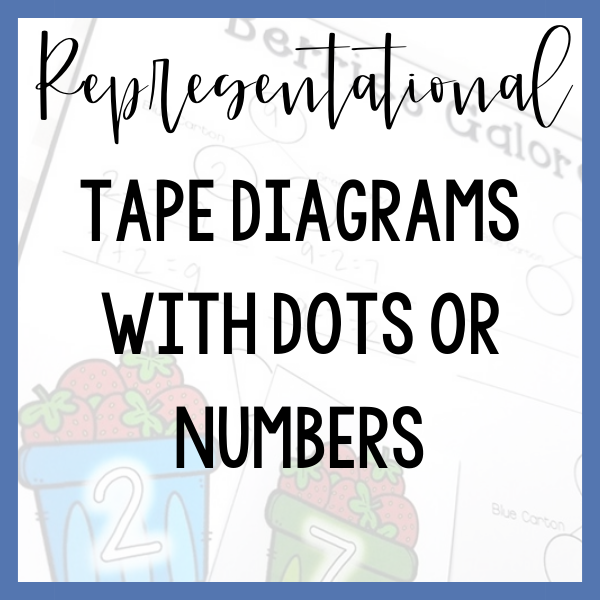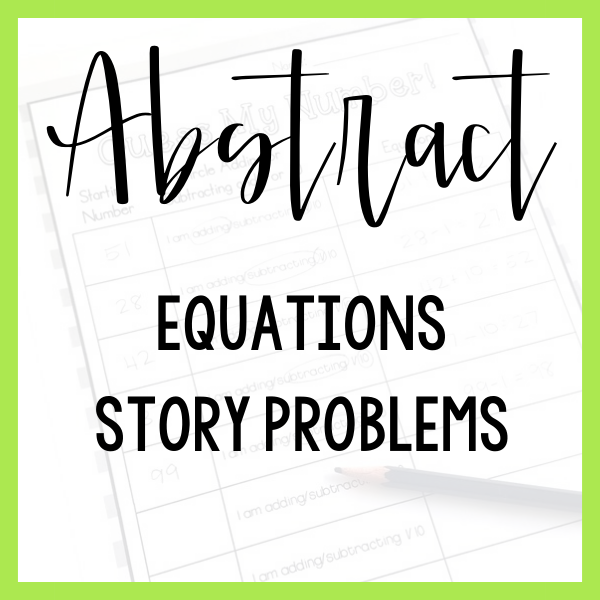# Multiplication and Division fact families

Third grade students are tasked with solving multiplication and division problems within 100. Students who understand the relationship between multiplication and division can more fluently solve both multiplication and division problems. Exploring fact families can help to highlight the inverse relationship of these two operations.The 5 examples listed are certainly not an exhaustive list of tools and lessons that could be used to teach this skill.

They are rather a sample progression from hands-on to abstract thinking!

Which step represents your students’ current level of understanding?

Try this:

Begin the lesson by telling students a story. 10 bees are buzzing around 2 beehives. They divide themselves evenly between the hives. How many bees are in each hive? Ask your students to model the scenario using the bee cubes and hives. Finally, ask your students to relate this scenario to a division equation.

Next, tell your students you want to use the same group of bees and put them back together. Could your students describe a multiplication equation that would put the 2 groups of 5 bees back together?

Your students have now found two equations relating 2, 5 and 10. Ask your students if there is a different way they could split the 10 bees into hives that still use these same numbers- 2, 5 and 10. Allow your students to each experiment with 10 cubes and an open supply of hives.

Connect your students knowledge of related facts using hands-on materials to a tape diagram filled with dots rather than numbers.

Try This:

Begin the lesson by telling your students the following story: I have 14 grocery items and I want them split into an equal number of shopping bags. Provide your students with a dry erase board and linking cubes and ask them to find one way that they could divide the 14 items evenly into grocery bags. Your students can draw circles to represent the bags and use linking cubes to represent the items in the bags.

Next, ask your students to draw a tape diagram that directly mirrors the bags and items they explored with the hands-on tools. Your students may, for example, draw 7 segments with 2 dots in each.

Ask your students to write a multiplication and division equation related to their diagram and to then further explore to find the other related equations, for example 2 segments with 7 dots in each.

Pairing hands-on, tape diagrams with dots and equations together allows your students to solidify their understanding of related equations.

It’s time to move away from hands-on materials! Instead, use what your students know (a tape diagram with pictures) and link to a new representation (a tape diagram with numbers).

Try This:

Begin this lesson with a story context “Mrs. Hoop has 20 kids in her gym class and she needs to break them up into two teams. I’m wondering how many students are on each team.”

Before using any methods to explore or solve this problem, ask your students if they have any predictions around how many students might be on each team. Look for students who are starting to recognize the relationship between multiplication and division. Record your students’ predictions on a white board.

Next, ask your students to draw a tape digram with dots in order to solve the problem.

Next, draw a second number bond directly beneath your first. Tell your students that as numbers get larger the dots may be difficult to keep track of, you can simply write numbers into the number bond to show the same information.

Ask your students if they can now generate a multiplication equation that also matches the tape diagrams. At this point you will have the equations 20 /2 = 10 and 2 x 10 = 20 listed.

Using the language “If we know 20/2 = 10, what other equation do we know?” or “If we know 2 x 20 = 20, what other equation do we know? Can help your students to generate related equations. Your students can then draw the related tape diagrams to confirm their thinking.

Supply your students with three related numbers (2, 5, 10 or 3, 4, 12 or 7, 3, 21 for example) . Ask your students to create a tape diagram that shows one way the parts could be put together. Allow each student to record this tape diagram on a white board.

Brainstorm a short story context that matches the tape diagram with a missing whole. Ex: Given the numbers 3, 4 and 12 the group may generate a tape diagram with 3 parts each having the number 4 inside with a total of 12. A related story might be “Three teams are running a relay race. There are 4 kids on each team. How many kids are running the race in all?”

Next, ask your students to write a multiplication and division equation directly related to their story context.

Use the sentence frame “If we know 3 x 4 = 12, what other multiplication equation do we know?” To support your students in finding additional related equations.

Your students can record a related tape diagram to confirm their thinking.

Begin the lesson by writing the number sentence 2 x 6 = 12  on a white board. Ask your students if they could tell a story that matches this number sentence.

Ask students if they could write a division equation around the same scenario. In this way, you are asking your students to write related facts with no scaffold other than a loose context. Ask your students to describe the context that matches their division number sentence.

For example, if the context was “Two boxes each have 6 crayons inside for a total of 12 crayons.” The matching division context would be “12 crayons divided into 2 boxes equals 6 crayons per box.”

Ask each student to produce a story and to write a related multiplication and division problem that match their scenario. Complete a gallery walk of the room with each student presenting their story and the related multiplication and division facts. You may have linking cubes available so students can demonstrate their scenario as well.

# Tools To USe